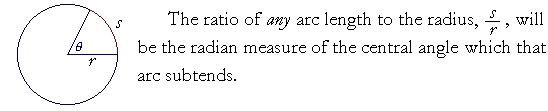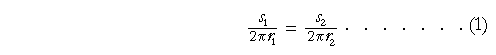14

# Arc Length

The definition of radian measure

s = rθ

The unit circleAn angle of 1 radian

Proof of the theoremIT IS CONVENTIONAL to let the letter s symbolize the length of an arc, which is called arc length. We say in geometry that an arc "subtends" an angle θ; literally, "stretches under."

Now the circumference of a circle is an arc length.  And the ratio of the circumference to the diameter is the basis of radian measure.  That ratio is the definition of π.

 π = CD .

Since D = 2r, then

 π = C2r

or,

 Cr = 2π .That ratio -- 2π -- of the circumference of a circle to the radius, is called the radian measure of 1 revolution, which are four right angles at the center.  The circumference subtends those four right angles.Radian measure of θ  = sr

Thus the radian measure is based on ratios -- numbers -- that are actually found in the circle.  The radian measure is a real number that indicates the ratio of a curved line to a straight, of an arc to the radius. For, the ratio of s to r does determine a unique central angle θ.

 Theorem. In any circles the same ratio of arc length to radius determines a unique central angle that the arcs subtend.Proportionally,if and only if

θ1 = θ2.

We will prove this theorem below.

 Example 1.  If s is 4 cm, and r is 5 cm, then the number 45 ,
 i.e. sr , is the radian measure of the central angle.

At that central angle, the arc is four fifths of the radius.

Example 2.   An angle of .75 radians means that the arc is three fourths of the radius.  s = .75r

Example 3.   In a circle whose radius is 10 cm, a central angle θ intercepts an arc of 8 cm.a)  What is the radian measure of that angle?

Answer.  According to the definition:

 θ  = sr = 810 =  .8

b)  At that same central angle θ, what is the arc length if the radius is 5 cm?Answer.  For a given central angle, the ratio of arc to radius is the same.  5 is half of 10.  Therefore the arc length will be half of 8:  4cm.

Example 4.

a)  At a central angle of 2.35 radians, what ratio has the arc to the radius?

Answer.  That number is the ratio.  The arc is 2.35 times the radius.

b)  In which quadrant of the circle does 2.35 radians fall?Answer.   Since π = 3.14, then π2 is half of that:  1.57.
 3π 2 = 3.14 + 1.57 = 4.71.

An angle of 2.35 radians, then, is greater than 1.57 but less that 3.14.  It falls in the second quadrant.

s = rθ

 c) If the radius is 10 cm, and the central angle is 2.35 radians, then how long is the arc?

Answer.  We let the definition of θ,

 θ  = sr

become a formula for finding s :

 s  =  rθ

Therefore,

s  = 10 × 2.35 = 23.5 cm

Because of the simplicity of that formula, radian measure is used exclusively in theoretical mathematics.

The unit circle

Since in any circle the same ratio of arc to radius determines a unique central angle, then for theoretical work we often use the unit circle, which is a circle of radius 1:  r = 1.In the unit circle, the radian measure is the length of the arc s.  The length of that arc is a real number x.

s = rθ = 1· x = x.

We can identify radian measure, then, as the length x of an arc of the unit circle.  And it is here that the term trigonometric "function" has its full meaning. For, corresponding to each real number x -- each radian measure, each arc -- there is a unique value of sin x, of cos x, and so on. The definition of a function is satisfied. (Topic 3 of Precalculus.)

Moreover, when we draw the graph of y = sin x (Topic 18), we can imagine the unit circle rolled out in both directions onto the x-axis, and in that way marking the coördinates π, 2π;, −π, −2π, and so on, on the x-axis.

Because radian measure can be identified as an arc, the inverse trigonometric functions have their names. "arcsin" is the arc -- the radian measure -- whose sine is a certain number.

 The ratio sin x  xIn the unit circle, the opposite side AB is sin x.

 sin x = AB 1 =  AB.

One of the main theorems in calculus concerns the ratio

 sin x   x

for very small values of x.  And we can see that when the point A on the circumference is very close to C -- that is, when the central angle AOC is very, very small -- then the opposite side AB will be virtually indistinguishable from the arc length AC.  That is,

 sin xx sin x   x1.

An angle of 1 radian

An angle of 1 radian refers to a central angle whose subtending arc is equal in length to the radius.That is often cited as the definition of radian measure.  Yet it remains to be proved that if an arc is equal to the radius in one circle, it will subtend the same central angle as an arc equal to the radius in another circle.  We cannot avoid the main theorem.

In addition, although it is possible to define an "angle of 1 radian," does such an angle actually exist? Is it possible to draw one -- a curved line equal to a straight line? Or is that but another example of fantasy mathematics?

See First Principles of Euclid's Elements, Commentary on the Definitions; see in particular that a definition asserts only how a word or a name will be used. It does not assert that what has been defined exists.

Problem 1.

 a)  At a central angle of π5 , approximately what ratio has

a)  the arc to the radius?  Take π3.

 The radian measure π5 is that ratio.  Taking π3,

then the arc is approximately three fifths of the radius.

b)  If the radius is 15 cm, approximately how long is the arc?

 s = rθ15· 35 = 9 cm

Problem 2.    In a circle whose radius is 4 cm, find the arc length intercepted by each of these angles.  Again, take π3.

 a) π4 s = rθ4· 34 = 3 cm
 b) π6 s = rθ4· 36 = 4· ½ = 2 cm
 c) 3π 2 s = rθ4· 3· 3  2 = 4· 92 = 2· 9 = 18 cm

d)  2π.  (Here, the arc length is the entire circumference!)

 s = rθ = 4· 2π4· 6 = 24 cm

Problem 3.   In which quadrant of the circle does each angle, measured in radians, fall? (See the figure above.)

 a)  θ = 2 2 radians are more than π2

but less than π.  (See the figure above.)  Therefore, θ = 2 falls in the second quadrant.

 b)  θ = 5 5 radians are more than 3π 2

but less than 2π.  (See the figure above.)  Therefore, θ = 5 falls in the fourth quadrant.

 c)  θ = 14 14 radians are more than 2 revolutions,

but slightly less than 2¼:  6.28 + 6.28 = 12.56.  (See the figure above.) Therefore, θ = 14 falls in the first quadrant.

Proof of the theorem

In any circles the same ratio of arc length to radius
determines a unique central angle that the arcs subtend;
and conversely, equal central angles determine the same ratio
of arc length to radius.Proportionally,if and only if

θ1 = θ2.

For,if and only ifNow 2πr is the circumference of each circle.  And each circumference is an "arc" that subtends four right angles at the center.But in the same circle, arcs have the same ratio to one another as the central angles they subtend.  (Theorem 16.)  Therefore,andTherefore, according to line (1),if and only if

θ1 = θ2.

Therefore, the same ratio of arc length to radius determines a unique central angle that the arcs subtend.  Which is what we wanted to prove.

Next Topic:  Analytic Trigonometry and the Unit Circle

Please make a donation to keep TheMathPage online.
Even \$1 will help.